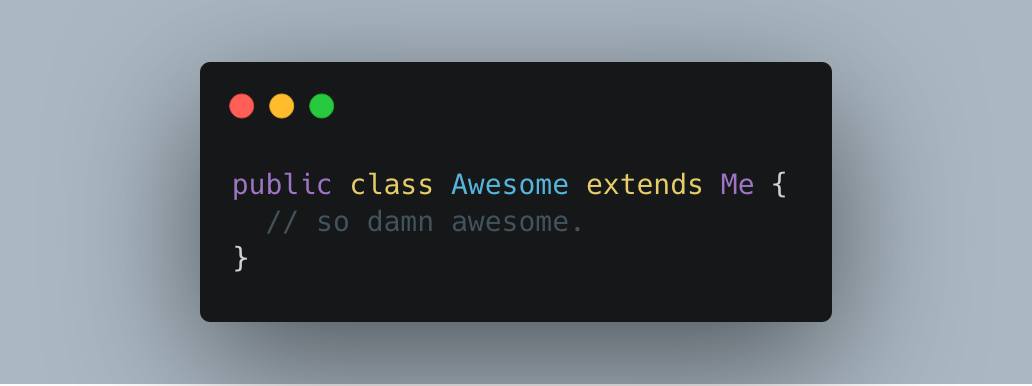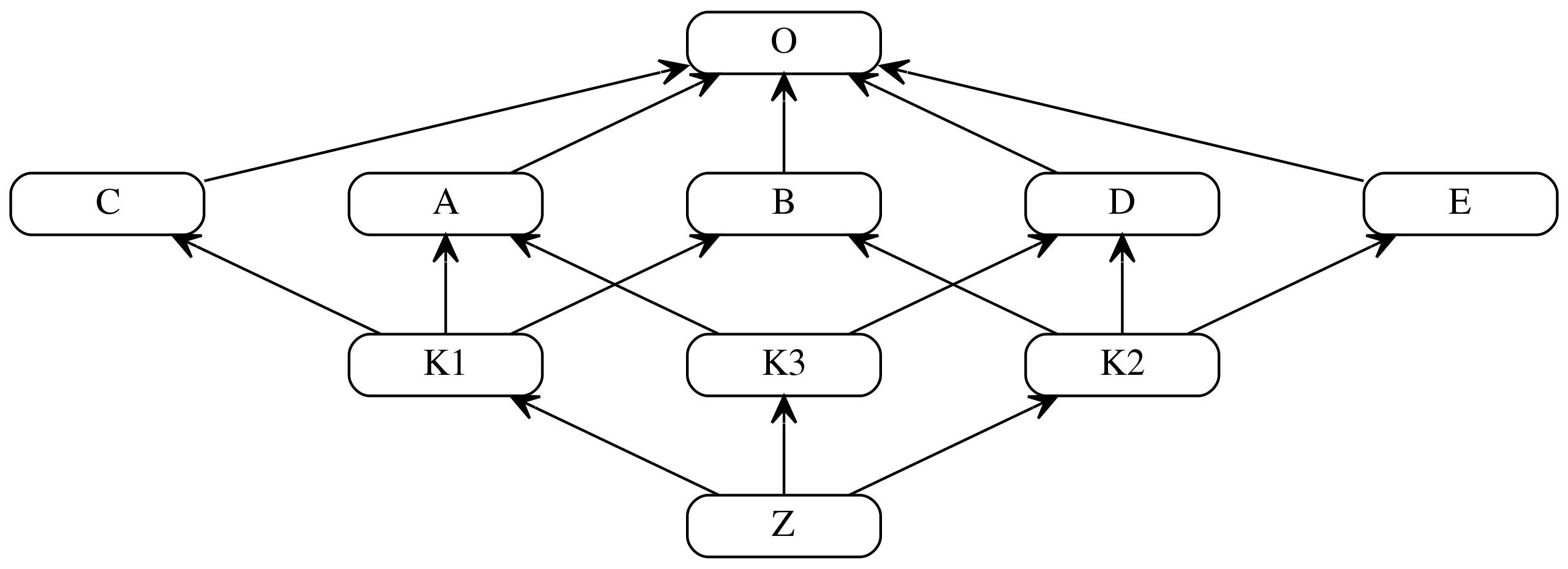# Python 多重继承问题之 MRO 和 C3 算法## 多重继承、MRO 及 C3算法的关系

﻿

C3算法实现了三种重要特性：

﻿

• 保持继承拓扑图的一致性。

• 保证局部优先原则（比如A继承C，C继承B，那么A读取父类方法，应该优先使用C的方法而不是B的方法）。

• 保证单调性原则（即子类不改变父类的方法搜索顺序）。

## 为什么采用C3算法

C3主要用于在多继承时判断继承调用的路径(来自于哪个类)。在Python2.3之前是基于深度优先算法，为了解决原来基于深度优先搜索算法不满足本地优先级，和单调性以及继承不清晰的问题，从Python2.3起应用了新的C3算法。 在Python官网的The Python 2.3 Method Resolution Order中作者举了例子，说明这一情况。

﻿

F=type('Food', (), {remember2buy:'spam'})E=type('Eggs', (F,), {remember2buy:'eggs'})G=type('GoodFood', (F,E), {})

﻿

## C3算法简介

class B(A)

﻿

class B(A1,A2,...,An)

mro(B) = [B] + merge(mro(A1), mro(A2),...,mro(An), [A1,A2,...,An])

﻿

merge操作是C3算法的核心，可以递归运算。

﻿

﻿

﻿

### 例子1

class A(object):passclass B(object):passclass C(object):passclass E(A,B):passclass F(B,C):passclass G(E,F):pass

mro(E) = [E] + merge(mro(A), mro(B), [A,B])       = [E] + merge([A,O], [B,O], [A,B])

mro(E) = [E,A] + merge([O], [B,O], [B])

mro(E) = [E,A,B] + merge([O], [O])       = [E,A,B,O]

mro(F) = [F] + merge(mro(B), mro(C), [B,C])           = [F] + merge([B,O], [C,O], [B,C])           = [F,B] + merge([O], [C,O], [C])           = [F,B,C] + merge([O], [O])           = [F,B,C,O]﻿mro(G) = [G] + merge(mro[E], mro[F], [E,F])           = [G] + merge([E,A,B,O], [F,B,C,O], [E,F])           = [G,E] + merge([A,B,O], [F,B,C,O], [F])           = [G,E,A] + merge([B,O], [F,B,C,O], [F])           = [G,E,A,F] + merge([B,O], [B,C,O])           = [G,E,A,F,B] + merge([O], [C,O])           = [G,E,A,F,B,C] + merge([O], [O])           = [G,E,A,F,B,C,O]

Wiki有一个Python版本的C3算法:

def c3MRO(cls):    if cls is object:        # 讨论假设顶层基类为object，递归终止        return [object]    # 构造C3-MRO算法的总式，递归开始    mergeList = [c3MRO(baseCls) for baseCls in cls.__bases__]    mergeList.append(list(cls.__bases__))    mro = [cls] + merge(mergeList)    return mrodef merge(inLists):    if not inLists:        # 若合并的内容为空，返回空list        # 配合下文的排除空list操作，递归终止        return []    # 遍历要合并的mro    for mroList in inLists:        # 取head        head = mroList        # 遍历要合并的mro（与外一层相同），检查尾中是否有head        ### 此处也遍历了被取head的mro，严格地来说不符合标准算法实现        ### 但按照多继承中地基础规则（一个类只能被继承一次），        ### head不可能在自己地尾中，无影响，若标准实现，反而增加开销        for cmpList in inLists[inLists.index(mroList) + 1:]:            if head in cmpList[1:]:                break        else:            # 筛选出好head            nextList = []            for mergeItem in inLists:                if head in mergeItem:                    mergeItem.remove(head)                if mergeItem:                    # 排除空list                    nextList.append(mergeItem)            # 递归开始            return [head] + merge(nextList)    else:        # 无好head，引发类型错误        raise TypeError

class A(object):passclass B(object):passclass C(object):passclass E(A,B):passclass F(B,C):passclass G(E,F):passprint([i.__name__ for i in c3MRO(G)]) ## ['G', 'E', 'A', 'F', 'B', 'C', 'object']

﻿

﻿

### 例子2

class Type(type):    def __repr__(cls):        return cls.__name__A = Type('A', (object,), {})B = Type('B', (object,), {})C = Type('C', (object,), {})D = Type('D', (object,), {})E = Type('E', (object,), {})K1 = Type('K1', (A, B, C), {})K2 = Type('K2', (D, B, E), {})K3 = Type('K3', (D, A), {})Z = Type('Z', (K1, K2,K3), {})

﻿﻿

﻿

print(Z.mro()) # [Z, K1, K2, K3, D, A, B, C, E, <class 'object'>]print([i.__name__ for i in c3MRO(Z)]) # ['Z', 'K1', 'K2', 'K3', 'D', 'A', 'B', 'C', 'E', 'object']

﻿

## 参考资料

Wiki百科——C3线性化

Python 2.3's use of C3 MRO

van Rossum, Guido. Method Resolution Order. The History of Python. 2010-06-23 [2018-01-18].

﻿## 评论